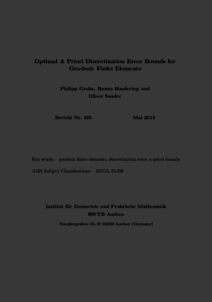Repository: Freie Universität Berlin, Math Department

# Optimal a priori discretization error bounds for geodesic finite elements

Grohs, P. and Hardering, H. and Sander, O. (2015) Optimal a priori discretization error bounds for geodesic finite elements. Foundations of Computational Mathematics, 15 (6). pp. 1357-1411. ISSN 1615-3375Preview

630kB

Official URL: http://dx.doi.org/10.1007/s10208-014-9230-z

## Abstract

We prove optimal bounds for the discretization error of geodesic finite elements for variational partial differential equations for functions that map into a nonlinear space. For this, we first generalize the well-known Céa lemma to nonlinear function spaces. In a second step, we prove optimal interpolation error estimates for pointwise interpolation by geodesic finite elements of arbitrary order. These two results are both of independent interest. Together they yield optimal a priori error estimates for a large class of manifold-valued variational problems. We measure the discretization error both intrinsically using an H1-type Finsler norm and with the H1-norm using embeddings of the codomain in a linear space. To measure the regularity of the solution, we propose a nonstandard smoothness descriptor for manifold-valued functions, which bounds additional terms not captured by Sobolev norms. As an application, we obtain optimal a priori error estimates for discretizations of smooth harmonic maps using geodesic finite elements, yielding the first high-order scheme for this problem.

Item Type: Article Geodesic finite elements, a priori error estimates, harmonic maps, high-order methods Mathematical and Computer Sciences > Mathematics > Numerical Analysis Department of Mathematics and Computer Science > Institute of Mathematics 1821 Ekaterina Engel 23 Feb 2016 09:29 03 Mar 2017 14:42

Repository Staff Only: item control page